# OpenGL Examples

Here are some OpenGL example programs, presented in an order in which new topics are added in each successive example. Many of these are modifications of programs in the OpenGL Red Book.

## Triangle• Introductory program; just a static picture of a colored triangle.
• Shows how to use GLUT.
• Has minimal structure: only `main()` and a display callback.
• Uses only the default viewing parameters (in fact, it never mentions viewing at all). This is an orthographic view volume with bounds of -1..1 in all three dimensions.
• Draws only using `glColor` and `glVertex` within `glBegin` and `glEnd` in the display callback.
• Uses only the `GL_POLYGON` drawing mode.
• Illustrates `glClear` and `glFlush`.
triangle.cpp
```// A simple introductory program; its main window contains a static picture
// of a triangle, whose three vertices are red, green and blue.  The program
// illustrates viewing with default viewing parameters only.

#ifdef __APPLE_CC__
#include <GLUT/glut.h>
#else
#include <GL/glut.h>
#endif

// Clears the current window and draws a triangle.
void display() {

// Set every pixel in the frame buffer to the current clear color.
glClear(GL_COLOR_BUFFER_BIT);

// Drawing is done by specifying a sequence of vertices.  The way these
// vertices are connected (or not connected) depends on the argument to
// glBegin.  GL_POLYGON constructs a filled polygon.
glBegin(GL_POLYGON);
glColor3f(1, 0, 0); glVertex3f(-0.6, -0.75, 0.5);
glColor3f(0, 1, 0); glVertex3f(0.6, -0.75, 0);
glColor3f(0, 0, 1); glVertex3f(0, 0.75, 0);
glEnd();

// Flush drawing command buffer to make drawing happen as soon as possible.
glFlush();
}

// Initializes GLUT, the display mode, and main window; registers callbacks;
// enters the main event loop.
int main(int argc, char** argv) {

// Use a single buffered window in RGB mode (as opposed to a double-buffered
// window or color-index mode).
glutInit(&argc, argv);
glutInitDisplayMode(GLUT_SINGLE | GLUT_RGB);

// Position window at (80,80)-(480,380) and give it a title.
glutInitWindowPosition(80, 80);
glutInitWindowSize(400, 300);
glutCreateWindow("A Simple Triangle");

// Tell GLUT that whenever the main window needs to be repainted that it
// should call the function display().
glutDisplayFunc(display);

// Tell GLUT to start reading and processing events.  This function
// never returns; the program only exits when the user closes the main
// window or kills the process.
glutMainLoop();
}
```

## Tetrahedron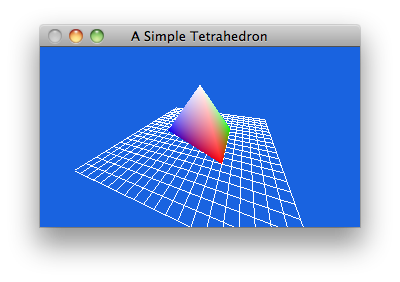• Displays a static picture of a tetrahedron sitting on a grid "floor".
• Sets up a viewing volume at the beginning of the program. Uses `glFrustum` in order to make a perspective projection.
• Shows that you have to define the viewing volume when the matrix mode is `GL_PROJECTION`.
• Draws the object in the display callback using `GL_LINES` and `GL_TRIANGLE_STRIP` modes.
• Defines the object at the origin then moves it INTO the view volume.
• Shows that the rotations and translations that move the object into the view volume must be performed when the matrix mode is `GL_MODELVIEW`.
• Shows that if you are not doing animation it is best to define the transformations that position and orient the object should be done only once, and not inside the display callback.
• Shows that transformations are cumulative.
• Shows that before setting up view volumes or object transformations, you usually want to do a `glLoadIdentity` or else transforamtions from previous manipulations will accumulate.
• Does backface culling as a very cheap way to do hidden surface removal. This does not work in all cases.
tetrahedron.cpp
```// This is a simple introductory program; its main window contains a static
// picture of a tetrahedron, whose top vertex is white and whose bottom
// vertices are red, green and blue.  The program illustrates viewing by
// defining an object at a convenient location, then transforming it so that
// it lies within the view volume.  This is a lousy way to do things (it's
// easier to use gluLookAt()), but it's nice to see how viewing is done at
// a very low level.

#ifdef __APPLE_CC__
#include <GLUT/glut.h>
#else
#include <GL/glut.h>
#endif

// Clears the window and draws the tetrahedron.  The tetrahedron is  easily
// specified with a triangle strip, though the specification really isn't very
void display() {
glClear(GL_COLOR_BUFFER_BIT);

// Draw a white grid "floor" for the tetrahedron to sit on.
glColor3f(1.0, 1.0, 1.0);
glBegin(GL_LINES);
for (GLfloat i = -2.5; i <= 2.5; i += 0.25) {
glVertex3f(i, 0, 2.5); glVertex3f(i, 0, -2.5);
glVertex3f(2.5, 0, i); glVertex3f(-2.5, 0, i);
}
glEnd();

// Draw the tetrahedron.  It is a four sided figure, so when defining it
// with a triangle strip we have to repeat the last two vertices.
glBegin(GL_TRIANGLE_STRIP);
glColor3f(1, 1, 1); glVertex3f(0, 2, 0);
glColor3f(1, 0, 0); glVertex3f(-1, 0, 1);
glColor3f(0, 1, 0); glVertex3f(1, 0, 1);
glColor3f(0, 0, 1); glVertex3f(0, 0, -1.4);
glColor3f(1, 1, 1); glVertex3f(0, 2, 0);
glColor3f(1, 0, 0); glVertex3f(-1, 0, 1);
glEnd();

glFlush();
}

// Sets up global attributes like clear color and drawing color, enables and
// initializes any needed modes (in this case we want backfaces culled), and
// sets up the desired projection and modelview matrices. It is cleaner to
// define these operations in a function separate from main().
void init() {

// Set the current clear color to sky blue and the current drawing color to
// white.
glClearColor(0.1, 0.39, 0.88, 1.0);
glColor3f(1.0, 1.0, 1.0);

// Tell the rendering engine not to draw backfaces.  Without this code,
// all four faces of the tetrahedron would be drawn and it is possible
// that faces farther away could be drawn after nearer to the viewer.
// Since there is only one closed polyhedron in the whole scene,
// eliminating the drawing of backfaces gives us the realism we need.
// THIS DOES NOT WORK IN GENERAL.
glEnable(GL_CULL_FACE);
glCullFace(GL_BACK);

// Set the camera lens so that we have a perspective viewing volume whose
// horizontal bounds at the near clipping plane are -2..2 and vertical
// bounds are -1.5..1.5.  The near clipping plane is 1 unit from the camera
// and the far clipping plane is 40 units away.
glMatrixMode(GL_PROJECTION);
glFrustum(-2, 2, -1.5, 1.5, 1, 40);

// Set up transforms so that the tetrahedron which is defined right at
// the origin will be rotated and moved into the view volume.  First we
// rotate 70 degrees around y so we can see a lot of the left side.
// Then we rotate 50 degrees around x to "drop" the top of the pyramid
// down a bit.  Then we move the object back 3 units "into the screen".
glMatrixMode(GL_MODELVIEW);
glTranslatef(0, 0, -3);
glRotatef(50, 1, 0, 0);
glRotatef(70, 0, 1, 0);
}

// Initializes GLUT, the display mode, and main window; registers callbacks;
// does application initialization; enters the main event loop.
int main(int argc, char** argv) {
glutInit(&argc, argv);
glutInitDisplayMode(GLUT_SINGLE | GLUT_RGB);
glutInitWindowPosition(80, 80);
glutInitWindowSize(800, 600);
glutCreateWindow("A Simple Tetrahedron");
glutDisplayFunc(display);
init();
glutMainLoop();
}
```

## Torus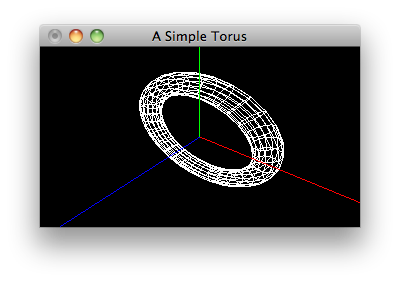• Display a static picture of a torus and the coordinate system axes.
• Instead of using a primitive GL function (such as `glOrtho` or `glFrustum`) to set the viewing volume, it instead uses `gluPerspective`. `gluPerspective` must be done when the matrix mode is `GL_PROJECTION`.
• Instead of using translates and rotates to position the objects into the view volume, it instead uses `gluLookAt` to position and orient the camera so that it is looking at the objects. `gluLookAt` must be done when the matrix mode is `GL_MODELVIEW`.
• Shows that the combination of `gluPerspective` and `gluLookAt` is considerably more intuitive than the more primitive mechanism of `glFrustum` and plain object transformations.
• Draws the torus using the fancy `glutWireTorus` function (which internally calls `glVertex` a zillion times).
torus.cpp
```// This is a simple introductory program; its main window contains a static
// picture of a torus.  The program illustrates viewing by choosing a camera
// setup with gluLookAt(), which is conceptually simpler than transforming
// objects to move into a predefined view volume.

#ifdef __APPLE_CC__
#include <GLUT/glut.h>
#else
#include <GL/glut.h>
#endif

// Clears the window and draws the torus.
void display() {

glClear(GL_COLOR_BUFFER_BIT);

// Draw a white torus of outer radius 3, inner radius 0.5 with 15 stacks
// and 30 slices.
glColor3f(1.0, 1.0, 1.0);
glutWireTorus(0.5, 3, 15, 30);

// Draw a red x-axis, a green y-axis, and a blue z-axis.  Each of the
// axes are ten units long.
glBegin(GL_LINES);
glColor3f(1, 0, 0); glVertex3f(0, 0, 0); glVertex3f(10, 0, 0);
glColor3f(0, 1, 0); glVertex3f(0, 0, 0); glVertex3f(0, 10, 0);
glColor3f(0, 0, 1); glVertex3f(0, 0, 0); glVertex3f(0, 0, 10);
glEnd();

glFlush();
}

// Sets up global attributes like clear color and drawing color, and sets up
// the desired projection and modelview matrices.
void init() {

// Set the current clear color to black and the current drawing color to
// white.
glClearColor(0.0, 0.0, 0.0, 1.0);
glColor3f(1.0, 1.0, 1.0);

// Set the camera lens to have a 60 degree (vertical) field of view, an
// aspect ratio of 4/3, and have everything closer than 1 unit to the
// camera and greater than 40 units distant clipped away.
glMatrixMode(GL_PROJECTION);
gluPerspective(60.0, 4.0/3.0, 1, 40);

// Position camera at (4, 6, 5) looking at (0, 0, 0) with the vector
// <0, 1, 0> pointing upward.
glMatrixMode(GL_MODELVIEW);
gluLookAt(4, 6, 5, 0, 0, 0, 0, 1, 0);
}

// Initializes GLUT, the display mode, and main window; registers callbacks;
// does application initialization; enters the main event loop.
int main(int argc, char** argv) {
glutInit(&argc, argv);
glutInitDisplayMode(GLUT_SINGLE | GLUT_RGB);
glutInitWindowPosition(80, 80);
glutInitWindowSize(800, 600);
glutCreateWindow("A Simple Torus");
glutDisplayFunc(display);
init();
glutMainLoop();
}
```

## Sierpinski2D• Draws 100000 points of a Sierpinski Gasket.
• Draws with GL_POINTS.
• Illustrates poor programming practice: the display callback contains long-running code. We get away with it here because we only have to generate 100000 points. The program will appear to hang if you wanted to draw, say, twenty million points.
• Ilustrates some C++, namely a `struct` with a member function.
• Note that the `glFlush` call is made after all 100000 points have been drawn, not after each point.
sierpinski2d.cpp
```// This program generates a Sierpinski gasket with 10000 points.

#ifdef __APPLE_CC__
#include <GLUT/glut.h>
#else
#include <GL/glut.h>
#endif
#include <cstdlib>

// A simple two-dimensional point class to make life easy.  It allows you to
// reference points with x and y coordinates instead of array indices) and
// encapsulates a midpoint function.
struct Point {
GLfloat x, y;
Point(GLfloat x = 0, GLfloat y = 0): x(x), y(y) {}
Point midpoint(Point p) {return Point((x + p.x) / 2.0, (y + p.y) / 2.0);}
};

// Draws a Sierpinski triangle with a fixed number of points. (Note that the
// number of points is kept fairly small because a display callback should
// NEVER run for too long.
void display() {

glClear(GL_COLOR_BUFFER_BIT);

static Point vertices[] = {Point(0, 0), Point(200, 500), Point(500, 0)};

// Compute and plot 100000 new points, starting (arbitrarily) with one of
// the vertices. Each point is halfway between the previous point and a
// randomly chosen vertex.
static Point p = vertices;
glBegin(GL_POINTS);
for (int k = 0; k < 100000; k++) {
p = p.midpoint(vertices[rand() % 3]);
glVertex2f(p.x, p.y);
}
glEnd();
glFlush();
}

// Performs application-specific initialization. Sets colors and sets up a
// simple orthographic projection.
void init() {

// Set a deep purple background and draw in a greenish yellow.
glClearColor(0.25, 0.0, 0.2, 1.0);
glColor3f(0.6, 1.0, 0.0);

// Set up the viewing volume: 500 x 500 x 1 window with origin lower left.
glMatrixMode(GL_PROJECTION);
glOrtho(0.0, 500.0, 0.0, 500.0, 0.0, 1.0);
}

// Initializes GLUT, the display mode, and main window; registers callbacks;
// does application initialization; enters the main event loop.
int main(int argc, char** argv) {
glutInit(&argc, argv);
glutInitDisplayMode (GLUT_SINGLE | GLUT_RGB);
glutInitWindowSize(500, 500);
glutInitWindowPosition(40, 40);
glutCreateWindow("Sierpinski Triangle");
glutDisplayFunc(display);
init();
glutMainLoop();
}
```

## Sierpinski3D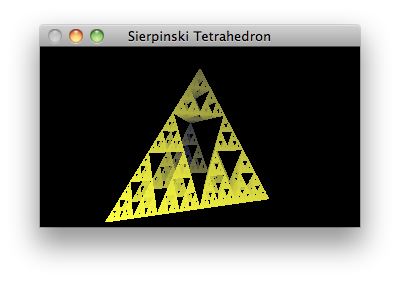• Draws points in the iteration of a 3-D Sierpinski Gasket for as long as the program runs.
• Illustrates the right way to perfom long-running drawing: in bursts during the idle time callback. The display callback doesn't do any drawing, other than clearing the windows (er, color buffer, I mean).
• Uses depth buffering so that points that would appear behind points already drawn will not themselves be drawn.
• Shows that depth buffering must be done by specifying the `GL_DEPTH` flag in `glutInitDisplayMode` and enable `GL_DEPTH_TEST`.
• Illustrates the reshape callback, which is absolutely essential for preserving the aspect ratio of the drawing.
• Shows that in order to preserve the aspect ration of the drawing, you set up the camera (in this case using `gluPerspective`) every time the window is reshaped. Note that the reshape callback is automatically called by GLUT shortly after the windowing system creates your window, so you don't need to force a call to a camera setup at the beginning of your program.
• Sets colors based on depth to make the picture look pretty cool.
sierpinski3d.cpp
```// This program is a modification and extension of an early program in Edward
// Angel's book, Interactive Computer Graphics: A top-down approach using
// OpenGL.  It plots the 3-D Sierpinski Tetrahedron using colors in a nice
// way.

#ifdef __APPLE_CC__
#include <GLUT/glut.h>
#else
#include <GL/glut.h>
#endif
#include <cstdlib>

// A simple three-dimensional point class to make life easy.  It allows you
// to reference points with x and y coordinates instead of array indices) and
// encapsulates a midpoint function.
struct Point {
GLfloat x, y, z;
Point(GLfloat x, GLfloat y, GLfloat z): x(x), y(y), z(z) {}
Point midpoint(Point p) {return Point((x+p.x)/2, (y+p.y)/2, (z+p.z)/2);}
};

// Handles reshape requests by setting the the viewport to exactly match the
// pixel coordinates, so we can draw in the whole window.  Then sets up a
// simple perspective viewing volume to ensure that the pyramid will never
// be distorted when the window is reshaped.  The particular settings chosen
// ensure that the vertical extent of the pyramid will always be visible.
void reshape(GLint w, GLint h) {
glViewport(0, 0, w, h);
glMatrixMode(GL_PROJECTION);
gluPerspective(100.0, GLfloat(w)/GLfloat(h), 10.0, 1500.0);
}

// Handles display requests.  All it has to do is clear the viewport because
// the real drawing is done in the idle callback.
void display() {
glClear(GL_COLOR_BUFFER_BIT | GL_DEPTH_BUFFER_BIT);
}

// Draws the "next 500 points".  The function contains locally the definitions
// of the vertices and the current point as static objects so that their values
// are retained between calls.
void generateMorePoints() {

// The tetrahedron has four vertices.  We also have to keep track of the
// current point during the plotting.
static Point vertices = {
Point(-250, -225, -200),
Point(-150, -225, -700),
Point(250, -225, -275),
Point(0, 450, -500)
};
static Point lastPoint = vertices;

// Here is the code to draw the "next 500 points".  The closer the point is
// to the camera, the brighter we make it.  The coloring technique is a
// quick hack which (unprofessionally) depends on the fact that the range
// of z-coordinates in the tetrahedron run from -200 to -700.  By adding
// 700 to any z-value and then dividing by 500, we get values in the range
// 0 to 1 - just perfect for coloring.
glBegin(GL_POINTS);
for (int i = 0; i <= 500; i++) {
lastPoint = lastPoint.midpoint(vertices[rand() % 4]);
GLfloat intensity = (700 + lastPoint.z) / 500.0;
glColor3f(intensity, intensity, 0.25);
glVertex3f(lastPoint.x, lastPoint.y, lastPoint.z);
}
glEnd();
glFlush();
}

// Performs application-specific initialization.  In this program we want to
// do depth buffering, which has to be explicitly enabled in OpenGL.
void init() {
glEnable(GL_DEPTH_TEST);
}

// Initializes GLUT, the display mode, and main window; registers callbacks;
// does application initialization; enters the main event loop.
int main(int argc, char** argv) {
glutInit(&argc, argv);
glutInitDisplayMode (GLUT_SINGLE | GLUT_RGB | GLUT_DEPTH);
glutInitWindowSize(500, 500);
glutInitWindowPosition(0, 0);
glutCreateWindow("Sierpinski Tetrahedron");
glutDisplayFunc(display);
glutReshapeFunc(reshape);
glutIdleFunc(generateMorePoints);
init();
glutMainLoop();
}
```

## Spinning Square• Animates a spinning square.
• Illustrates animation with double buffering. This requires passing `GLUT_DOUBLE` to `glutInitDisplayMode`, generating the parameters for the next animation frame in the timer callback which posts a redisplay request, and finishing off the display callback with a call to `glutSwapBuffers`.
• Shows that it is better to accumulate transformation parameters, rather than the transformations themselves, when doing cyclical animation.
• Illustrates the mouse callback.
• Shows that callbacks can be removed. In this case, the idle callback gets removed.
• Shows that maintaining the aspect ratio of a drawing when an orthographic projection is used is a bigger pain than doing so with a perspective projection using `gluPerspective`.
spinningsquare.cpp
```// This program demonstrates double buffering for flicker-free animation.
// It spins a white square on a black background.  It comes from Chapter 1
// of the OpenGL Programming Guide, but I made some minor changes, and did
// the animation properly, using timers, not the idle function.  Start the
// animation with the left mouse button and stop it with the right.

#ifdef __APPLE_CC__
#include <GLUT/glut.h>
#else
#include <GL/glut.h>
#endif

// Set this to true to animate.
static bool spinning = true;

// This is the number of frames per second to render.
static const int FPS = 60;

// This global variable keeps track of the current orientation of the square.
// It is better to maintain the "state" of the animation globally rather
// than trying to successively accumulate transformations.  Over a period of
// time the approach of accumulating transformation matrices generally
// degrades due to rounding errors.
static GLfloat currentAngleOfRotation = 0.0;

// Handles the window reshape event by first ensuring that the viewport fills
// the entire drawing surface.  Then we use a simple orthographic projection
// with a logical coordinate system ranging from -50..50 in the smaller of
// the width or height, and scale the larger dimension to make the whole
// window isotropic.
void reshape(GLint w, GLint h) {
glViewport(0, 0, w, h);
GLfloat aspect = (GLfloat)w / (GLfloat)h;
glMatrixMode(GL_PROJECTION);
if (w <= h) {
// width is smaller, go from -50 .. 50 in width
glOrtho(-50.0, 50.0, -50.0/aspect, 50.0/aspect, -1.0, 1.0);
} else {
// height is smaller, go from -50 .. 50 in height
glOrtho(-50.0*aspect, 50.0*aspect, -50.0, 50.0, -1.0, 1.0);
}
}

// Handles the display callback as follows: first clears the window, then draws
// a 50 x 50 rectangle centered at the origin and rotated the correct number
// of degrees around the vector <0,0,1>.  This function ends with a
// 'glutSwapBuffers' call because when the display mode is double buffered,
// drawing takes place on the back buffer; we have to call glutSwapBuffers()
// to show what we have drawn.
void display() {
glClear(GL_COLOR_BUFFER_BIT);
glMatrixMode(GL_MODELVIEW);
glRotatef(currentAngleOfRotation, 0.0, 0.0, 1.0);
glRectf(-25.0, -25.0, 25.0, 25.0);
glFlush();
glutSwapBuffers();
}

// Handles the timer by incrementing the angle of rotation and requesting the
// window to display again, provided the program is in the spinning state.
// Since the timer function is only called once, it sets the same function to
// be called again.
void timer(int v) {
if (spinning) {
currentAngleOfRotation += 1.0;
if (currentAngleOfRotation > 360.0) {
currentAngleOfRotation -= 360.0;
}
glutPostRedisplay();
}
glutTimerFunc(1000/FPS, timer, v);
}

// Handles mouse events as follows: when the left button is pressed, generate
// new animation frames while the application is idle; when the right button
// is pressed, remove any idle-time callbacks, thus stopping the animation.
void mouse(int button, int state, int x, int y) {
if (button == GLUT_LEFT_BUTTON && state == GLUT_DOWN) {
spinning = true;
} else if (button == GLUT_RIGHT_BUTTON && state == GLUT_DOWN) {
spinning = false;
}
}

// Initializes GLUT, the display mode, and main window; registers callbacks;
// enters the main event loop.
int main(int argc, char** argv) {
glutInit(&argc, argv);
glutInitDisplayMode(GLUT_DOUBLE | GLUT_RGB);
glutInitWindowPosition(80, 80);
glutInitWindowSize(800, 500);
glutCreateWindow("Spinning Square");
glutReshapeFunc(reshape);
glutDisplayFunc(display);
glutTimerFunc(100, timer, 0);
glutMouseFunc(mouse);
glutMainLoop();
}
```

## Interactive Robot Arm• Lets you move a robot arm, with a shoulder and an elbow, with the arrow keys.
• Is a very simple program that manages to illustrate the very important concept of hierarchical modeling.
• Shows that to draw an object in the context of a "parent" object you arrange drawing and matrix manipulation code in a certain order.
• Illustrates `glPushMatrix` and `glPopMatrix` (note how this makes `wireBox` "reusable" in any context).
• Shows that the keyboard callback, for keys other than normal printable characters, is called the "special" callback in GLUT.
robotarm.cpp
```// This program is from the OpenGL Programming Guide.  It shows a robot arm
// that you can rotate by pressing the arrow keys.

#ifdef __APPLE_CC__
#include <GLUT/glut.h>
#else
#include <GL/glut.h>
#endif

// The robot arm is specified by (1) the angle that the upper arm makes
// relative to the x-axis, called shoulderAngle, and (2) the angle that the
// lower arm makes relative to the upper arm, called elbowAngle.  These angles
// are adjusted in 5 degree increments by a keyboard callback.
static int shoulderAngle = 0, elbowAngle = 0;

// Handles the keyboard event: the left and right arrows bend the elbow, the
// up and down keys bend the shoulder.
void special(int key, int, int) {
switch (key) {
case GLUT_KEY_LEFT: (elbowAngle += 5) %= 360; break;
case GLUT_KEY_RIGHT: (elbowAngle -= 5) %= 360; break;
case GLUT_KEY_UP: (shoulderAngle += 5) %= 360; break;
case GLUT_KEY_DOWN: (shoulderAngle -= 5) %= 360; break;
default: return;
}
glutPostRedisplay();
}

// wireBox(w, h, d) makes a wireframe box with width w, height h and
// depth d centered at the origin.  It uses the GLUT wire cube function.
// The calls to glPushMatrix and glPopMatrix are essential here; they enable
// this function to be called from just about anywhere and guarantee that
// the glScalef call does not pollute code that follows a call to myWireBox.
void wireBox(GLdouble width, GLdouble height, GLdouble depth) {
glPushMatrix();
glScalef(width, height, depth);
glutWireCube(1.0);
glPopMatrix();
}

// Displays the arm in its current position and orientation.  The whole
// function is bracketed by glPushMatrix and glPopMatrix calls because every
// time we call it we are in an "environment" in which a gluLookAt is in
// effect.  (Note that in particular, replacing glPushMatrix with
// glLoadIdentity makes you lose the camera setting from gluLookAt).
void display() {

glClear(GL_COLOR_BUFFER_BIT);
glMatrixMode(GL_MODELVIEW);
glPushMatrix();

// Draw the upper arm, rotated shoulder degrees about the z-axis.  Note that
// the thing about glutWireBox is that normally its origin is in the middle
// of the box, but we want the "origin" of our box to be at the left end of
// the box, so it needs to first be shifted 1 unit in the x direction, then
// rotated.
glRotatef((GLfloat)shoulderAngle, 0.0, 0.0, 1.0);
glTranslatef(1.0, 0.0, 0.0);
wireBox(2.0, 0.4, 1.0);

// Now we are ready to draw the lower arm.  Since the lower arm is attached
// to the upper arm we put the code here so that all rotations we do are
// relative to the rotation that we already made above to orient the upper
// arm.  So, we want to rotate elbow degrees about the z-axis.  But, like
// before, the anchor point for the rotation is at the end of the box, so
// we translate <1,0,0> before rotating.  But after rotating we have to
// position the lower arm at the end of the upper arm, so we have to
// translate it <1,0,0> again.
glTranslatef(1.0, 0.0, 0.0);
glRotatef((GLfloat)elbowAngle, 0.0, 0.0, 1.0);
glTranslatef(1.0, 0.0, 0.0);
wireBox(2.0, 0.4, 1.0);

glPopMatrix();
glFlush();
}

// Handles the reshape event by setting the viewport so that it takes up the
// whole visible region, then sets the projection matrix to something reason-
// able that maintains proper aspect ratio.
void reshape(GLint w, GLint h) {
glViewport(0, 0, w, h);
glMatrixMode(GL_PROJECTION);
gluPerspective(65.0, GLfloat(w)/GLfloat(h), 1.0, 20.0);
}

// Perfroms application specific initialization: turn off smooth shading,
// sets the viewing transformation once and for all.  In this application we
// won't be moving the camera at all, so it makes sense to do this.
void init() {
glMatrixMode(GL_MODELVIEW);
gluLookAt(1,2,8, 0,0,0, 0,1,0);
}

// Initializes GLUT, the display mode, and main window; registers callbacks;
// does application initialization; enters the main event loop.
int main(int argc, char** argv) {
glutInit(&argc, argv);
glutInitDisplayMode(GLUT_SINGLE | GLUT_RGB);
glutInitWindowPosition(80, 80);
glutInitWindowSize(800, 600);
glutCreateWindow("Robot Arm");
glutDisplayFunc(display);
glutReshapeFunc(reshape);
glutSpecialFunc(special);
init();
glutMainLoop();
}
```

## Color Cube Flyby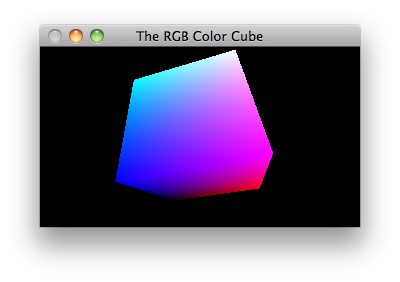• Is our first example of a "flyby" program.
• Performs animation by moving the camera on a closed orbit around a stationary object, which just happens to be the standard RGB color cube.
• Shows that on flybys, you set the camera shape (`gluPerspective` in `GL_PROJECTION` mode) in the reshape callback, but set the camera position and orientation (`gluLookAt` in `GL_MODELVIEW` mode) for every frame you draw.
• Draws with `GL_QUADS` and the moderately ugly `glVertex3iv` function.
• Animates with a timer doing a delay of 16.6667 ms between finishing the drawing of one frame and starting the next.
colorcube.cpp
```// This program is a flyby around the RGB color cube.  One intersting note
// is that because the cube is a convex polyhedron and it is the only thing
// in the scene, we can render it using backface culling only. i.e., there
// is no need for a depth buffer.

#ifdef __APPLE_CC__
#include <GLUT/glut.h>
#else
#include <GL/glut.h>
#endif
#include <cmath>

// The cube has opposite corners at (0,0,0) and (1,1,1), which are black and
// white respectively.  The x-axis is the red gradient, the y-axis is the
// green gradient, and the z-axis is the blue gradient.  The cube's position
// and colors are fixed.
namespace Cube {

const int NUM_VERTICES = 8;
const int NUM_FACES = 6;

GLint vertices[NUM_VERTICES] = {
{0, 0, 0}, {0, 0, 1}, {0, 1, 0}, {0, 1, 1},
{1, 0, 0}, {1, 0, 1}, {1, 1, 0}, {1, 1, 1}};

GLint faces[NUM_FACES] = {
{1, 5, 7, 3}, {5, 4, 6, 7}, {4, 0, 2, 6},
{3, 7, 6, 2}, {0, 1, 3, 2}, {0, 4, 5, 1}};

GLfloat vertexColors[NUM_VERTICES] = {
{0.0, 0.0, 0.0}, {0.0, 0.0, 1.0}, {0.0, 1.0, 0.0}, {0.0, 1.0, 1.0},
{1.0, 0.0, 0.0}, {1.0, 0.0, 1.0}, {1.0, 1.0, 0.0}, {1.0, 1.0, 1.0}};

void draw() {
for (int i = 0; i < NUM_FACES; i++) {
for (int j = 0; j < 4; j++) {
glColor3fv((GLfloat*)&vertexColors[faces[i][j]]);
glVertex3iv((GLint*)&vertices[faces[i][j]]);
}
}
glEnd();
}
}

// Display and Animation. To draw we just clear the window and draw the cube.
// Because our main window is double buffered we have to swap the buffers to
// make the drawing visible. Animation is achieved by successively moving our
// camera and drawing. The function nextAnimationFrame() moves the camera to
// the next point and draws. The way that we get animation in OpenGL is to
// register nextFrame as the idle function; this is done in main().
void display() {
glClear(GL_COLOR_BUFFER_BIT);
Cube::draw();
glFlush();
glutSwapBuffers();
}

// We'll be flying around the cube by moving the camera along the orbit of the
// curve u->(8*cos(u), 7*cos(u)-1, 4*cos(u/3)+2).  We keep the camera looking
// at the center of the cube (0.5, 0.5, 0.5) and vary the up vector to achieve
// a weird tumbling effect.
void timer(int v) {
static GLfloat u = 0.0;
u += 0.01;
gluLookAt(8*cos(u), 7*cos(u)-1, 4*cos(u/3)+2, .5, .5, .5, cos(u), 1, 0);
glutPostRedisplay();
glutTimerFunc(1000/60.0, timer, v);
}

// When the window is reshaped we have to recompute the camera settings to
// match the new window shape.  Set the viewport to (0,0)-(w,h).  Set the
// camera to have a 60 degree vertical field of view, aspect ratio w/h, near
// clipping plane distance 0.5 and far clipping plane distance 40.
void reshape(int w, int h) {
glViewport(0, 0, w, h);
glMatrixMode(GL_PROJECTION);
gluPerspective(60.0, GLfloat(w) / GLfloat(h), 0.5, 40.0);
glMatrixMode(GL_MODELVIEW);
}

// Application specific initialization:  The only thing we really need to do
// is enable back face culling because the only thing in the scene is a cube
// which is a convex polyhedron.
void init() {
glEnable(GL_CULL_FACE);
glCullFace(GL_BACK);
}

// The usual main for a GLUT application.
int main(int argc, char** argv) {
glutInit(&argc, argv);
glutInitDisplayMode(GLUT_DOUBLE | GLUT_RGB);
glutInitWindowSize(500, 500);
glutCreateWindow("The RGB Color Cube");
glutReshapeFunc(reshape);
glutTimerFunc(100, timer, 0);
glutDisplayFunc(display);
init();
glutMainLoop();
}
```

## Comet Ride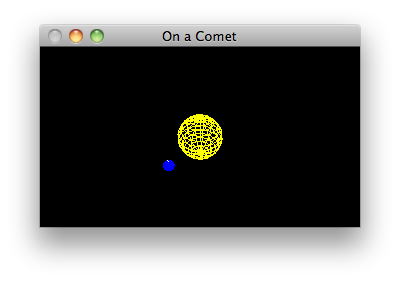• Animates the sun and earth from the point of view of a comet.
• Is another flyby with a predefined flight path.
• Flies around a scene with multiple objects which themselves are moving.
• The objects are the sun and the earth, drawn as wire spheres.
• Animation again uses simplistic timer functions.
cometride.cpp
```// This program does a little solar system simulation from the point of view
// of a comet.  Here there is a yellow sun and a blue  planet; the planet
// spins on its own axis and it rotates around the sun.  The viewer is
// sitting on a comet and is always facing the sun.

#ifdef __APPLE_CC__
#include <GLUT/glut.h>
#else
#include <GL/glut.h>
#endif
#include <cmath>

// In the GLUT library someone put the polar regions on the z-axis - yech!!
// We fixed it so that they are on the y-axis.  We do this by rotating -90
// degrees about the x-axis which brings (0,0,1) to (0,1,0).
void myWireSphere(GLfloat radius, int slices, int stacks) {
glPushMatrix();
glRotatef(-90.0, 1.0, 0.0, 0.0);
glPopMatrix();
}

// In our solar system simulation we keep track of the current "year" and
// current "day" in global variables.  Actually we just make the planet go
// around the sun in increments of 5 degrees (the "year") and rotate the
// planet on its own axis in increments of 10 degrees (the "day").
static int year = 0, day = 0;

// As usual, the routine to display the current state of the system is
// bracketed with a clearing of the window and a glFlush call.  Immediately
// within those calls the drawing code itself is bracketed by pushing and
// popping the current transformation.  And also as usual, we are assuming the
// current matrix mode is GL_MODELVIEW.  We finish with a SwapBuffers call
// because we'll animate.
void display() {
glClear(GL_COLOR_BUFFER_BIT | GL_DEPTH_BUFFER_BIT);
glPushMatrix();

// Draw sun: a yellow sphere of radius 1 centered at the origin.
glColor3f(1.0, 1.0, 0.0);
myWireSphere(1.0, 15, 15);

// Draw planet: a blue sphere of radius 0.2, 2 units away from sun, with
// a white "pole" for its axis.
glRotatef((GLfloat)year, 0.0, 1.0, 0.0);
glTranslatef (2.0, 0.0, 0.0);
glRotatef((GLfloat)day, 0.0, 1.0, 0.0);
glColor3f(0.0, 0.0, 1.0);
myWireSphere(0.2, 15, 15);
glColor3f(1, 1, 1);
glBegin(GL_LINES);
glVertex3f(0, -0.3, 0);
glVertex3f(0, 0.3, 0);
glEnd();

glPopMatrix();
glFlush();
glutSwapBuffers();
}

// Viewing routines.  Basically the camera is sitting on a comet orbiting
// the sun with a "year" 8 times that of the planet.  To animate we have
// the function nextAnimationFrame() registered as the idle function.  It
// increments the value of u (to "move" the camera), ticks off another
// portion of a day and portion of a year, then reorients the camera and
// refreshes the display.
static GLfloat u = 0.0;                 // curve parameter for comet pos
static GLfloat du = 0.1;                // amt to increment u each frame

void timer(int v) {
u += du;
day = (day + 1) % 360;
year = (year + 2) % 360;
gluLookAt(20*cos(u/8.0)+12,5*sin(u/8.0)+1,10*cos(u/8.0)+2, 0,0,0, 0,1,0);
glutPostRedisplay();
glutTimerFunc(1000/60, timer, v);
}

// As usual we reset the projection transformation whenever the window is
// reshaped.  This is done (of course) by setting the current matrix mode
// to GL_PROJECTION and then setting the matrix.  It is easiest to use the
// perspective-projection-making matrix from the GL utiltiy library.  Here
// we set a perspective camera with a 60-degree vertical field of view,
// an aspect ratio to perfectly map into the system window, a near clipping
// plane distance of 1.0 and a far clipping distance of 40.0.  The last
// thing done is to reset the current matrix mode to GL_MODELVIEW, as
// that is expected in all the calls to display().
void reshape(GLint w, GLint h) {
glViewport(0, 0, w, h);
glMatrixMode(GL_PROJECTION);
gluPerspective(60.0, (GLfloat)w/(GLfloat)h, 1.0, 40.0);
glMatrixMode(GL_MODELVIEW);
}

// The usual main() for a GLUT application.
int main(int argc, char** argv) {
glutInit(&argc, argv);
glutInitDisplayMode (GLUT_DOUBLE | GLUT_RGB | GLUT_DEPTH);
glutInitWindowSize(800, 600);
glutCreateWindow("On a Comet");
glutDisplayFunc(display);
glutReshapeFunc(reshape);
glutTimerFunc(100, timer, 0);
glEnable(GL_DEPTH_TEST);
glutMainLoop();
}
```

## Lit Solids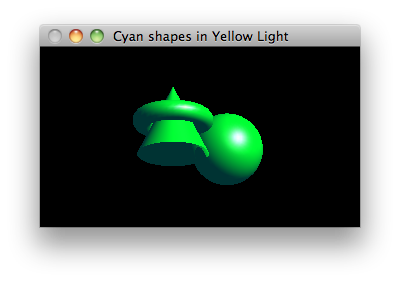• Displays a static picture of three cyan solids lit by a single yellow light source.
• Shows that lighting, like depth buffering, is something that must be enabled.
• Uses ambient, diffuse and specular parameters for lighting.
• Uses a directional light source, as opposed to a point light source.
• Illustrates three of the GLUT easy shape functions.
• Each object is independently moved into the viewing volume, showing the importance of `glPushMatrix` and `glPopMatrix`.
litsolids.cpp
```// This program shows three cyan objects illuminated with a single yellow
// light source.  It illustrates several of the lighting parameters.

#ifdef __APPLE_CC__
#include <GLUT/glut.h>
#else
#include <GL/glut.h>
#endif

// Clears the window and depth buffer and draws three solids.
//
// The solids are placed so that they either sit or float above the x-z plane;
// therefore note one of the first things that is done is to rotate the whole
// scene 20 degrees about x to turn the top of the scene toward the viewer.
// This lets the viewer see how the torus goes around the cone.
void display() {
glClear(GL_COLOR_BUFFER_BIT | GL_DEPTH_BUFFER_BIT);
glMatrixMode(GL_MODELVIEW);
glPushMatrix();

// Rotate the scene so we can see the tops of the shapes.
glRotatef(-20.0, 1.0, 0.0, 0.0);

// Make a torus floating 0.5 above the x-z plane.  The standard torus in
// the GLUT library is, perhaps surprisingly, a stack of circles which
// encircle the z-axis, so we need to rotate it 90 degrees about x to
// get it the way we want.
glPushMatrix();
glTranslatef(-0.75, 0.5, 0.0);
glRotatef(90.0, 1.0, 0.0, 0.0);
glutSolidTorus(0.275, 0.85, 16, 40);
glPopMatrix();

// Make a cone.  The standard cone "points" along z; we want it pointing
// along y, hence the 270 degree rotation about x.
glPushMatrix();
glTranslatef(-0.75, -0.5, 0.0);
glRotatef(270.0, 1.0, 0.0, 0.0);
glutSolidCone(1.0, 2.0, 70, 12);
glPopMatrix();

// Add a sphere to the scene.
glPushMatrix();
glTranslatef(0.75, 0.0, -1.0);
glutSolidSphere(1.0, 30, 30);
glPopMatrix();

glPopMatrix();
glFlush();
}

// We don't want the scene to get distorted when the window size changes, so
// we need a reshape callback.  We'll always maintain a range of -2.5..2.5 in
// the smaller of the width and height for our viewbox, and a range of -10..10
// for the viewbox depth.
void reshape(GLint w, GLint h) {
glViewport(0, 0, w, h);
glMatrixMode(GL_PROJECTION);
GLfloat aspect = GLfloat(w) / GLfloat(h);
if (w <= h) {
// width is smaller, so stretch out the height
glOrtho(-2.5, 2.5, -2.5/aspect, 2.5/aspect, -10.0, 10.0);
} else {
// height is smaller, so stretch out the width
glOrtho(-2.5*aspect, 2.5*aspect, -2.5, 2.5, -10.0, 10.0);
}
}

// Performs application specific initialization.  It defines lighting
// parameters for light source GL_LIGHT0: black for ambient, yellow for
// diffuse, white for specular, and makes it a directional source
// shining along <-1, -1, -1>.  It also sets a couple material properties
// to make cyan colored objects with a fairly low shininess value.  Lighting
// and depth buffer hidden surface removal are enabled here.
void init() {
GLfloat black[] = { 0.0, 0.0, 0.0, 1.0 };
GLfloat yellow[] = { 1.0, 1.0, 0.0, 1.0 };
GLfloat cyan[] = { 0.0, 1.0, 1.0, 1.0 };
GLfloat white[] = { 1.0, 1.0, 1.0, 1.0 };
GLfloat direction[] = { 1.0, 1.0, 1.0, 0.0 };

glMaterialfv(GL_FRONT, GL_AMBIENT_AND_DIFFUSE, cyan);
glMaterialfv(GL_FRONT, GL_SPECULAR, white);
glMaterialf(GL_FRONT, GL_SHININESS, 30);

glLightfv(GL_LIGHT0, GL_AMBIENT, black);
glLightfv(GL_LIGHT0, GL_DIFFUSE, yellow);
glLightfv(GL_LIGHT0, GL_SPECULAR, white);
glLightfv(GL_LIGHT0, GL_POSITION, direction);

glEnable(GL_LIGHTING);                // so the renderer considers light
glEnable(GL_LIGHT0);                  // turn LIGHT0 on
glEnable(GL_DEPTH_TEST);              // so the renderer considers depth
}

// The usual application statup code.
int main(int argc, char** argv) {
glutInit(&argc, argv);
glutInitDisplayMode(GLUT_SINGLE | GLUT_RGB | GLUT_DEPTH);
glutInitWindowPosition(80, 80);
glutInitWindowSize(800, 600);
glutCreateWindow("Cyan Shapes in Yellow Light");
glutReshapeFunc(reshape);
glutDisplayFunc(display);
init();
glutMainLoop();
}
```

## Phases of the Moon• Simulates the phases of the moon with a simple animation.
• Draws with display lists!
• Shows that display lists are defined once, but called many times, and hence are virtually necessary in all nontrivial animations that require high performance.
• Puts the lighting definitions in the display lists for cool effects.
moon.cpp
```// In this program the camera orbits a lit moon to simulate the phases of the
// moon.

#ifdef __APPLE_CC__
#include <GLUT/glut.h>
#else
#include <GL/glut.h>
#endif
#include <cmath>

// A class for the moon.  A moon is really just an OpenGL display list.  The
// display list is created with create(), and the draw() method calls it.
// The reason we don't create the display list in the constructor is that
// clients may want to declare moon objects before the call to initializing
// OpenGL.
//
// The moon is a sphere of radius 1 centered at the origin, built from 25
// slices and 25 stacks, lit with GL_LIGHT0 as a directional light pointing
// along <1,1,1>.
class Moon {
int displayListId;
public:
void create() {
displayListId = glGenLists(1);
glNewList(displayListId, GL_COMPILE);
GLfloat direction[] = {-1.0, -1.0, -1.0, 0.0};
glLightfv(GL_LIGHT0, GL_POSITION, direction);
glutSolidSphere(1.0, 25, 25);
glEndList();
}
void draw() {
glCallList(displayListId);
}
};

// The one and only moon.
static Moon moon;

// An orbiter is an object that flies on a circle of a certain radius on the
// xz plane.  You supply the radius at construction time.
class Orbiter {
double u;
public:
void advance(double delta) {u += delta;}
void getPosition(double& x, double& y, double& z) {
y = 0;
}
};

// The one and only orbiter.
static Orbiter orbiter(5.0);

// Clears the window (and the depth buffer) and draws the moon as viewed from
// the current position of the orbiter.
void display() {
glClear(GL_COLOR_BUFFER_BIT | GL_DEPTH_BUFFER_BIT);
glMatrixMode(GL_MODELVIEW);
glPushMatrix();
double x, y, z;
orbiter.getPosition(x, y, z);
gluLookAt(x, y, z, 0.0, 0.0, 0.0, 0.0, 1.0, 0.0);
moon.draw();
glPopMatrix();
glutSwapBuffers();
}

// Advances the orbiter and requests to draw the next frame.
void timer(int v) {
glutPostRedisplay();
glutTimerFunc(1000/60, timer, v);
}

// reshape() fixes up the projection matrix so that we always see a sphere
void reshape(GLint w, GLint h) {
glViewport(0, 0, w, h);
glMatrixMode(GL_PROJECTION);
gluPerspective(40.0, GLfloat(w) / GLfloat(h), 1.0, 10.0);
}

// Enables depth testing, enables lighting for a bright yellowish diffuse
// light, and creates a moon.
void init() {
glEnable(GL_DEPTH_TEST);
GLfloat yellow[] = {1.0, 1.0, 0.5, 1.0};
glLightfv(GL_LIGHT0, GL_DIFFUSE, yellow);
glEnable(GL_LIGHTING);
glEnable(GL_LIGHT0);
moon.create();
}

// The usual application code.
int main(int argc, char** argv) {
glutInit(&argc, argv);
glutInitDisplayMode(GLUT_DOUBLE | GLUT_RGB | GLUT_DEPTH);
glutInitWindowPosition(80, 80);
glutInitWindowSize(500, 500);
glutCreateWindow("The Moon");
glutDisplayFunc(display);
glutTimerFunc(100, timer, 0);
glutReshapeFunc(reshape);
init();
glutMainLoop();
}
```

## Bouncing Balls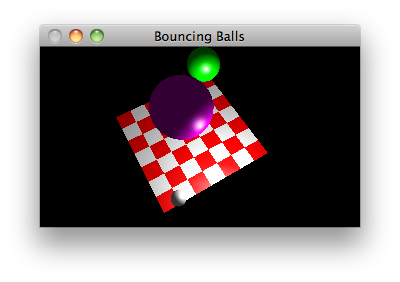• Shows balls bouncing on a checkerboard.
• Features a point light source.
• Moves the camera in response to key presses.
bouncingballs.cpp
```// This application shows balls bouncing on a checkerboard, with no respect
// for the laws of Newtonian Mechanics.  There's a little spotlight to make
// the animation interesting, and arrow keys move the camera for even more
// fun.

#ifdef __APPLE_CC__
#include <GLUT/glut.h>
#else
#include <GL/glut.h>
#endif
#include <cmath>

// Colors
GLfloat WHITE[] = {1, 1, 1};
GLfloat RED[] = {1, 0, 0};
GLfloat GREEN[] = {0, 1, 0};
GLfloat MAGENTA[] = {1, 0, 1};

// A camera.  It moves horizontally in a circle centered at the origin of
// radius 10.  It moves vertically straight up and down.
class Camera {
double theta;      // determines the x and z positions
double y;          // the current y position
double dTheta;     // increment in theta for swinging the camera around
double dy;         // increment in y for moving the camera up/down
public:
Camera(): theta(0), y(3), dTheta(0.04), dy(0.2) {}
double getX() {return 10 * cos(theta);}
double getY() {return y;}
double getZ() {return 10 * sin(theta);}
void moveRight() {theta += dTheta;}
void moveLeft() {theta -= dTheta;}
void moveUp() {y += dy;}
void moveDown() {if (y > dy) y -= dy;}
};

// A ball.  A ball has a radius, a color, and bounces up and down between
// a maximum height and the xz plane.  Therefore its x and z coordinates
// are fixed.  It uses a lame bouncing algorithm, simply moving up or
// down by 0.05 units at each frame.
class Ball {
GLfloat* color;
double maximumHeight;
double x;
double y;
double z;
int direction;
public:
Ball(double r, GLfloat* c, double h, double x, double z):
y(h), x(x), z(z) {
}
void update() {
y += direction * 0.05;
if (y > maximumHeight) {
y = maximumHeight; direction = -1;
} else if (y < radius) {
y = radius; direction = 1;
}
glPushMatrix();
glMaterialfv(GL_FRONT, GL_AMBIENT_AND_DIFFUSE, color);
glTranslated(x, y, z);
glPopMatrix();
}
};

// A checkerboard class.  A checkerboard has alternating red and white
// squares.  The number of squares is set in the constructor.  Each square
// is 1 x 1.  One corner of the board is (0, 0) and the board stretches out
// along positive x and positive z.  It rests on the xz plane.  I put a
// spotlight at (4, 3, 7).
class Checkerboard {
int displayListId;
int width;
int depth;
public:
Checkerboard(int width, int depth): width(width), depth(depth) {}
double centerx() {return width / 2;}
double centerz() {return depth / 2;}
void create() {
displayListId = glGenLists(1);
glNewList(displayListId, GL_COMPILE);
GLfloat lightPosition[] = {4, 3, 7, 1};
glLightfv(GL_LIGHT0, GL_POSITION, lightPosition);
glNormal3d(0, 1, 0);
for (int x = 0; x < width - 1; x++) {
for (int z = 0; z < depth - 1; z++) {
glMaterialfv(GL_FRONT, GL_AMBIENT_AND_DIFFUSE,
(x + z) % 2 == 0 ? RED : WHITE);
glVertex3d(x, 0, z);
glVertex3d(x+1, 0, z);
glVertex3d(x+1, 0, z+1);
glVertex3d(x, 0, z+1);
}
}
glEnd();
glEndList();
}
void draw() {
glCallList(displayListId);
}
};

// Global variables: a camera, a checkerboard and some balls.
Checkerboard checkerboard(8, 8);
Camera camera;
Ball balls[] = {
Ball(1, GREEN, 7, 6, 1),
Ball(1.5, MAGENTA, 6, 3, 4),
Ball(0.4, WHITE, 5, 1, 7)
};

// Application-specific initialization: Set up global lighting parameters
// and create display lists.
void init() {
glEnable(GL_DEPTH_TEST);
glLightfv(GL_LIGHT0, GL_DIFFUSE, WHITE);
glLightfv(GL_LIGHT0, GL_SPECULAR, WHITE);
glMaterialfv(GL_FRONT, GL_SPECULAR, WHITE);
glMaterialf(GL_FRONT, GL_SHININESS, 30);
glEnable(GL_LIGHTING);
glEnable(GL_LIGHT0);
checkerboard.create();
}

// Draws one frame, the checkerboard then the balls, from the current camera
// position.
void display() {
glClear(GL_COLOR_BUFFER_BIT | GL_DEPTH_BUFFER_BIT);
gluLookAt(camera.getX(), camera.getY(), camera.getZ(),
checkerboard.centerx(), 0.0, checkerboard.centerz(),
0.0, 1.0, 0.0);
checkerboard.draw();
for (int i = 0; i < sizeof balls / sizeof(Ball); i++) {
balls[i].update();
}
glFlush();
glutSwapBuffers();
}

// On reshape, constructs a camera that perfectly fits the window.
void reshape(GLint w, GLint h) {
glViewport(0, 0, w, h);
glMatrixMode(GL_PROJECTION);
gluPerspective(40.0, GLfloat(w) / GLfloat(h), 1.0, 150.0);
glMatrixMode(GL_MODELVIEW);
}

// Requests to draw the next frame.
void timer(int v) {
glutPostRedisplay();
glutTimerFunc(1000/60, timer, v);
}

// Moves the camera according to the key pressed, then ask to refresh the
// display.
void special(int key, int, int) {
switch (key) {
case GLUT_KEY_LEFT: camera.moveLeft(); break;
case GLUT_KEY_RIGHT: camera.moveRight(); break;
case GLUT_KEY_UP: camera.moveUp(); break;
case GLUT_KEY_DOWN: camera.moveDown(); break;
}
glutPostRedisplay();
}

// Initializes GLUT and enters the main loop.
int main(int argc, char** argv) {
glutInit(&argc, argv);
glutInitDisplayMode(GLUT_DOUBLE | GLUT_RGB | GLUT_DEPTH);
glutInitWindowPosition(80, 80);
glutInitWindowSize(800, 600);
glutCreateWindow("Bouncing Balls");
glutDisplayFunc(display);
glutReshapeFunc(reshape);
glutSpecialFunc(special);
glutTimerFunc(100, timer, 0);
init();
glutMainLoop();
}
```

## Flight Simulator

• Is a flyby that is controlled by user interaction (the previous flybys moved the camera along a predermined orbit).
• Makes use of several supporting modules:
• geometry.h: a module defining basic classes for points, lines, planes and rays.
• ship.h: a class representing the control of an interactive spaceship: you can set its position, speed, orientation, and direction in different ways. Uses real mathematics!
• landscape.h and landscape.cpp: a class for fractal landscapes.
• cockpit.h: a class with a `draw` method which renders a ship's cockpit, sort of.
• Illustrates rendering a scene in which different parts of the scene use different projections and views.

## Fish Bitmaps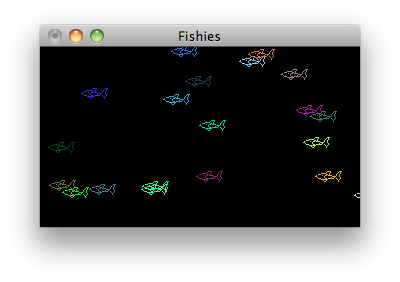• A first program illustrating bitmaps, drawing twenty instances of a fish bitmap at random positions in a window.
• Uses only the default settings for all pixel store settings, so the program is not very fancy.
fish.cpp
```// Shows a trivial use of OpenGL bitmaps, so trivial in fact, that it uses
// nothing but the default settings for glPixelStore*.

#ifdef __APPLE_CC__
#include <GLUT/glut.h>
#else
#include <GL/glut.h>
#endif
#include <cstdlib>

// A fish bitmap, size is 27x11, but all rows must have a multiple of 8 bits,
// so we define it like it is 32x11.
GLubyte fish[] = {
0x00, 0x60, 0x01, 0x00,
0x00, 0x90, 0x01, 0x00,
0x03, 0xf8, 0x02, 0x80,
0x1c, 0x37, 0xe4, 0x40,
0x20, 0x40, 0x90, 0x40,
0xc0, 0x40, 0x78, 0x80,
0x41, 0x37, 0x84, 0x80,
0x1c, 0x1a, 0x04, 0x80,
0x03, 0xe2, 0x02, 0x40,
0x00, 0x11, 0x01, 0x40,
0x00, 0x0f, 0x00, 0xe0,
};

// Return a random float in the range 0.0 to 1.0.
GLfloat randomFloat() {
return (GLfloat)rand() / RAND_MAX;
}

// On reshape, uses an orthographic projection with world coordinates ranging
// from 0 to 1 in the x and y directions, and -1 to 1 in z.
void reshape(int width, int height) {
glViewport(0, 0, width, height);
glMatrixMode(GL_PROJECTION);
gluOrtho2D(0, 1, 0, 1);
}

// Clears the window then plots 20 fish bitmaps in random colors at random
// positions.
void display() {
glClear(GL_COLOR_BUFFER_BIT);
for (int i = 0; i < 20; i++) {
glColor3f(randomFloat(), randomFloat(), randomFloat());
glRasterPos3f(randomFloat(), randomFloat(), 0.0);
glBitmap(27, 11, 0, 0, 0, 0, fish);
}
glFlush();
}

// The usual main() for a GLUT application.
int main(int argc, char **argv) {
glutInit(&argc, argv);
glutInitDisplayMode(GLUT_RGB | GLUT_SINGLE);
glutInitWindowSize(400, 300);
glutCreateWindow("Fishies");
glutReshapeFunc(reshape);
glutDisplayFunc(display);
glutMainLoop();
}
```

## Checkered Triangles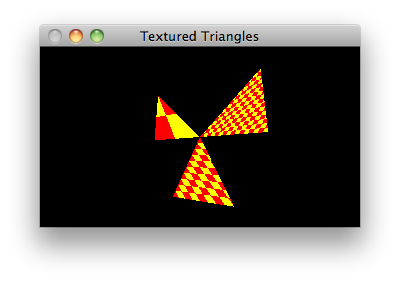• A first program illustration of textures.
• Illustrates how to construt textures "by hand"
• Illustrates some pixel store and texture settings.
checkeredtriangles.cpp
```// This application is a trivial illustration of texture mapping.  It draws
// several triangles, each with a texture mapped on to it.  The same texture
// is used for each triangle, but the mappings vary quite a bit so it looks as
// if each triangle has a different texture.

#ifdef __APPLE_CC__
#include <GLUT/glut.h>
#else
#include <GL/glut.h>
#endif
#include <cstdlib>

// Define a 2 x 2 red and yellow checkered pattern using RGB colors.
#define red {0xff, 0x00, 0x00}
#define yellow {0xff, 0xff, 0x00}
#define magenta {0xff, 0, 0xff}
GLubyte texture[] = {
red, yellow,
yellow, red,
};

// Fixes up camera and remaps texture when window reshaped.
void reshape(int width, int height) {
glViewport(0, 0, width, height);
glMatrixMode(GL_PROJECTION);
gluPerspective(80, GLfloat(width)/height, 1, 40);
glMatrixMode(GL_MODELVIEW);
gluLookAt(2, -1, 5, 0, 0, 0, 0, 1, 0);
glEnable(GL_TEXTURE_2D);
glPixelStorei(GL_UNPACK_ALIGNMENT, 1);
glTexImage2D(GL_TEXTURE_2D,
0,                    // level 0
3,                    // use only R, G, and B components
2, 2,                 // texture has 2x2 texels
0,                    // no border
GL_RGB,               // texels are in RGB format
GL_UNSIGNED_BYTE,     // color components are unsigned bytes
texture);
glTexParameteri(GL_TEXTURE_2D, GL_TEXTURE_MIN_FILTER, GL_NEAREST);
glTexParameteri(GL_TEXTURE_2D, GL_TEXTURE_MAG_FILTER, GL_NEAREST);
}

// Draws three textured triangles.  Each triangle uses the same texture,
// but the mappings of texture coordinates to vertex coordinates is
// different in each triangle.
void display() {
glClear(GL_COLOR_BUFFER_BIT);
glBegin(GL_TRIANGLES);
glTexCoord2f(0.5, 1.0);    glVertex2f(-3, 3);
glTexCoord2f(0.0, 0.0);    glVertex2f(-3, 0);
glTexCoord2f(1.0, 0.0);    glVertex2f(0, 0);

glTexCoord2f(4, 8);        glVertex2f(3, 3);
glTexCoord2f(0.0, 0.0);    glVertex2f(0, 0);
glTexCoord2f(8, 0.0);      glVertex2f(3, 0);

glTexCoord2f(5, 5);        glVertex2f(0, 0);
glTexCoord2f(0.0, 0.0);    glVertex2f(-1.5, -3);
glTexCoord2f(4, 0.0);      glVertex2f(1.5, -3);
glEnd();
glFlush();
}

// Initializes GLUT and enters the main loop.
int main(int argc, char** argv) {
glutInit(&argc, argv);
glutInitDisplayMode(GLUT_SINGLE | GLUT_RGB);
glutInitWindowSize(520, 390);
glutCreateWindow("Textured Triangles");
glutDisplayFunc(display);
glutReshapeFunc(reshape);
glutMainLoop();
}
```